## Example Questions

### Example Question #126 : Integers

Of a group of 335 graduating high school atheletes, 106 played basketball, 137 ran track and field, and 51 participated in swimming. What is the maximum number of students that did both track and field and swimming upon graduation?

335

106

51

55

157

51

Explanation:

Simply recognize that logically, the participation of either sport is non-exclusive, that is, just because people took track and field does not necessarily mean they did not take swimming as well. As such, those 51 who took swimming could have all potentially done track and field, which means all 51 students.

### Example Question #127 : Integers

Of 300 students, 120 are enrolled in math club, 150 are enrolled in chess club, and 100 are enrolled in both. How many students are not members of either club?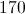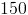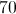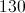Explanation:

There are 120 students in the math club and 150 students in the chess club, for a total membership of 270. However, 100 students are in both clubs, which means they are counted twice. You simply subtract 100 from 270, which will give you a total of 170 different students participating in both clubs. This means that the remaining 130 students do not participate in either club.

### Example Question #128 : Integers

Choose the answer which best solves the following equation: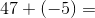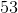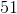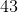Explanation:

When adding integers, one needs to pay close attention to the sign.  When you add a negative integer, it's the same thing as subtracting that integer.  Therefore: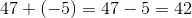### Example Question #129 : Integers

Choose the answer below which best solves the following equation: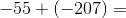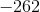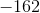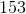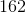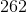Explanation:

The sum of any two negative numbers will be negative.  Remember, also, that adding a negative number is like subtracting it.  Therefore: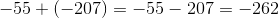Tired of practice problems?

Try live online GRE prep today.